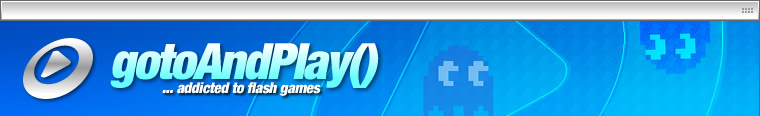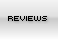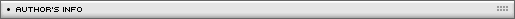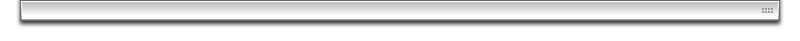3D throw ball
[ July 22, 2004 ] by Eric Lin
Intermediate 3D scripting for Flash part 5/5:
Learn how to create an interactive 3D bouncing ball

If you have experience in making 3d flash movie, the scripts are nearly self -evident. No secrets here. You can close this page now. It is a good practice for beginner to create a 3d movie like this. The first step is learning the scripting about throw a ball in 2 D plane. Usually we do it by a parabolar equation. It is scripted as "acceleration".

```onClipEvent (load) {
xSpeed = 5;
ySpeed = -40;
gravity = 1.5;
x = _x;
y = _y;
}
onClipEvent (enterFrame) {
ySpeed += gravity;
x += xSpeed;
y += ySpeed;
_x = x;
_y = y;
}
```

This is enough for throw a ball. Then we will add bounce when the ball hit the ground. I will not talk about this now.
Notice my coding style. I manipulate x, y instead of _x, _y. Only at the last line, I make _x=x;_y=y; to show it on the screen.

This way, the codes are the same of 3D movie. Only the last lines _x=x;_y=y; will change. The second step is borrow a 3d equations, and make your Xspeed into Zspeed, because we want throw to the Z direction.

Now apply the 3d equation to get _x, _y, _z according to the x,y,z;
The simplest 3d equation is in the form of scale=M/(N+z); or scale=zoom*(100/100+z);

```zoomZ = 100;
shiftX = 0;
shiftY =0;
z0=100;
PZ = {x:50, y:250};

function f3dscale (z) {
var scale = zoomZ*z0/(z0+z);
return scale;
}
function f3dPoint (x, y, z) {
var x3d = (x+shiftX)*f3dscale(z)/100+PZ.x;
var y3d = (y+shiftY)*f3dscale(z)/100+PZ.y;
var pt = {x:x3d, y:y3d};
return pt;
}

So, for a ball with x,y,z

_x=f3dPoint(x,y,z).x;
_y=f3dPoint(x,y,z).y;
_xscale=_yscale=f3dscale(z);
```

Download the source code of this prototypeName: Eric Lin Location: Taiwan Age: 46 Flash experience: Since Flash 5, about 4 years Job: neurosurgeon (a doctor who operates people's brain) Website: http://ericlin2.tripod.com/| Homepage | News | Games | Articles | Multiplayer Central | Reviews | Spotlight | Forums | Info | Links | Contact us | Advertise | Credits || www.smartfoxserver.com | www.gotoandplay.biz | www.openspace-engine.com | gotoAndPlay() v 3.0.0 -- (c)2003-2008 gotoAndPlay() Team -- P.IVA 03121770048